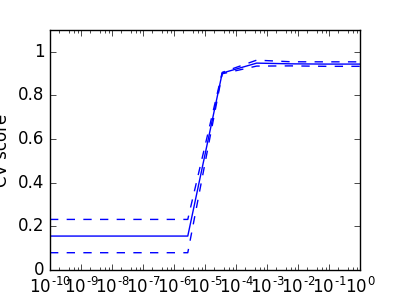# Model selection: choosing estimators and their parameters¶

## Score, and cross-validated scores¶

As we have seen, every estimator exposes a `score` method that can judge the quality of the fit (or the prediction) on new data. Bigger is better.

```>>> from sklearn import datasets, svm
>>> X_digits = digits.data
>>> y_digits = digits.target
>>> svc = svm.SVC(C=1, kernel='linear')
>>> svc.fit(X_digits[:-100], y_digits[:-100]).score(X_digits[-100:], y_digits[-100:])
0.97999999999999998
```

To get a better measure of prediction accuracy (which we can use as a proxy for goodness of fit of the model), we can successively split the data in folds that we use for training and testing:

```>>> import numpy as np
>>> X_folds = np.array_split(X_digits, 3)
>>> y_folds = np.array_split(y_digits, 3)
>>> scores = list()
>>> for k in range(3):
...     # We use 'list' to copy, in order to 'pop' later on
...     X_train = list(X_folds)
...     X_test  = X_train.pop(k)
...     X_train = np.concatenate(X_train)
...     y_train = list(y_folds)
...     y_test  = y_train.pop(k)
...     y_train = np.concatenate(y_train)
...     scores.append(svc.fit(X_train, y_train).score(X_test, y_test))
>>> print(scores)
[0.93489148580968284, 0.95659432387312182, 0.93989983305509184]
```

This is called a `KFold` cross-validation.

## Cross-validation generators¶

Scikit-learn has a collection of classes which can be used to generate lists of train/test indices for popular cross-validation strategies.

They expose a `split` method which accepts the input dataset to be split and yields the train/test set indices for each iteration of the chosen cross-validation strategy.

This example shows an example usage of the `split` method.

```>>> from sklearn.model_selection import KFold, cross_val_score
>>> X = ["a", "a", "b", "c", "c", "c"]
>>> k_fold = KFold(n_splits=3)
>>> for train_indices, test_indices in k_fold.split(X):
...      print('Train: %s | test: %s' % (train_indices, test_indices))
Train: [2 3 4 5] | test: [0 1]
Train: [0 1 4 5] | test: [2 3]
Train: [0 1 2 3] | test: [4 5]
```

The cross-validation can then be performed easily:

```>>> kfold = KFold(n_splits=3)
>>> [svc.fit(X_digits[train], y_digits[train]).score(X_digits[test], y_digits[test])
...          for train, test in k_fold.split(X_digits)]
[0.93489148580968284, 0.95659432387312182, 0.93989983305509184]
```

The cross-validation score can be directly calculated using the `cross_val_score` helper. Given an estimator, the cross-validation object and the input dataset, the `cross_val_score` splits the data repeatedly into a training and a testing set, trains the estimator using the training set and computes the scores based on the testing set for each iteration of cross-validation.

By default the estimator’s `score` method is used to compute the individual scores.

Refer the metrics module to learn more on the available scoring methods.

```>>> cross_val_score(svc, X_digits, y_digits, cv=k_fold, n_jobs=-1)
array([ 0.93489149,  0.95659432,  0.93989983])
```

n_jobs=-1 means that the computation will be dispatched on all the CPUs of the computer.

Alternatively, the `scoring` argument can be provided to specify an alternative scoring method.

```>>> cross_val_score(svc, X_digits, y_digits, cv=k_fold,
...                 scoring='precision_macro')
array([ 0.93969761,  0.95911415,  0.94041254])
```

Cross-validation generators

 `KFold` (n_splits, shuffle, random_state) `StratifiedKFold` (n_iter, test_size, train_size, random_state) `GroupKFold` (n_splits, shuffle, random_state) Splits it into K folds, trains on K-1 and then tests on the left-out. Same as K-Fold but preserves the class distribution within each fold. Ensures that the same group is not in both testing and training sets.
 `ShuffleSplit` (n_iter, test_size, train_size, random_state) `StratifiedShuffleSplit` `GroupShuffleSplit` Generates train/test indices based on random permutation. Same as shuffle split but preserves the class distribution within each iteration. Ensures that the same group is not in both testing and training sets.
 `LeaveOneGroupOut` () `LeavePGroupsOut` (p) `LeaveOneOut` () Takes a group array to group observations. Leave P groups out. Leave one observation out.
 `LeavePOut` (p) `PredefinedSplit` Leave P observations out. Generates train/test indices based on predefined splits.

ExerciseOn the digits dataset, plot the cross-validation score of a `SVC` estimator with an linear kernel as a function of parameter `C` (use a logarithmic grid of points, from 1 to 10).

```
import numpy as np
from sklearn.model_selection import cross_val_score
from sklearn import datasets, svm

X = digits.data
y = digits.target

svc = svm.SVC(kernel='linear')
C_s = np.logspace(-10, 0, 10)
```

## Grid-search and cross-validated estimators¶

### Cross-validated estimators¶

Cross-validation to set a parameter can be done more efficiently on an algorithm-by-algorithm basis. This is why, for certain estimators, scikit-learn exposes Cross-validation: evaluating estimator performance estimators that set their parameter automatically by cross-validation:

```>>> from sklearn import linear_model, datasets
>>> lasso = linear_model.LassoCV()
>>> X_diabetes = diabetes.data
>>> y_diabetes = diabetes.target
>>> lasso.fit(X_diabetes, y_diabetes)
LassoCV(alphas=None, copy_X=True, cv=None, eps=0.001, fit_intercept=True,
max_iter=1000, n_alphas=100, n_jobs=1, normalize=False, positive=False,
precompute='auto', random_state=None, selection='cyclic', tol=0.0001,
verbose=False)
>>> # The estimator chose automatically its lambda:
>>> lasso.alpha_
0.01229...
```

These estimators are called similarly to their counterparts, with ‘CV’ appended to their name.

Exercise

On the diabetes dataset, find the optimal regularization parameter alpha.

Bonus: How much can you trust the selection of alpha?

```
from sklearn import datasets
from sklearn.linear_model import LassoCV
from sklearn.linear_model import Lasso
from sklearn.model_selection import KFold
from sklearn.model_selection import cross_val_score